# Forth and back

Car goes from point A to point B at speed 78 km/h and back at 82 km/h. If went there and back at speed 81 km/h journey would take five minutes less. What is the distance between points A and B?

Correct result:

x =  256.9821 km

#### Solution:

x = 78a
x = 82b
2x = 81(a+b-5/60)

x = 78•a
x = 82•b
2•x = 81•(a+b-5/60)

78a-x = 0
82b-x = 0
4860a+4860b-120x = 405

a = 369/112 ≈ 3.294643
b = 351/112 ≈ 3.133929
x = 14391/56 ≈ 256.982143

Our linear equations calculator calculates it.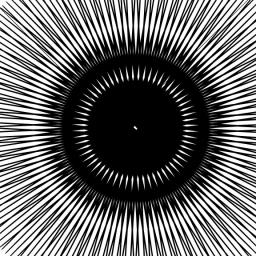We would be pleased if you find an error in the word problem, spelling mistakes, or inaccuracies and send it to us. Thank you!Tips to related online calculators
Looking for calculator of harmonic mean?
Looking for a statistical calculator?
Do you have a linear equation or system of equations and looking for its solution? Or do you have quadratic equation?
Do you want to convert velocity (speed) units?
Do you want to convert time units like minutes to seconds?

## Next similar math problems:

• Car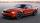Car goes from point A to point B at speed 86 km/h and back 53 km/h. If they goes there and back at speed 67 km/h trip would take 10 minutes shorter. What is distance between points A and B?
• Two citiesThe car goes from city A to city B at an average speed of 70 km/h, back at an average speed of 50 km/h. If it goes to B and back at an average speed of 60 km/h, the whole ride would take 8 minutes less. What is the distance between cities A and B?
• Tourist Jirka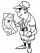Distance between the points A and B is 13.5 km. Jirka went from point A to point B unknown speed and for an unknown period of time. Back to the point A went slower by 3 km/h which means that went 20 minutes more. How long Jirka took the return journey?
• Steamship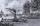Between the two ports on the river is going steamship. The journey there and back takes 8:42. Downstream steamship traveling at 20 km/h, upstream 9 km/h. What is the distance between the ports?
• Distance between two citiesCar went from A to B 4h. On the way back, the car was up 15km / h faster. The return trip took 48 minutes. Shorter than the way there. Find the distance of the cities A and B.
• Pedestrian up-down hill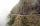Pedestrian goes for a walk first at plane at 4 km/h, then uphill 3 km/h. Then it is in the middle of the route, turns back and goes downhill at speed 6 km/h. Total walk was 6 hours. How many kilometers went pedestrian?
• Journey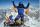The road from A to B measures 11.5 km. Firstly up the hill, then by level plane and then downhill. Tourist goes uphill at 3 km/h, on the plane 4 km/h and downhill 5 km/h. From point A to B went 2h 54 min back 3h 6 min. How long is the segment of level pla
• FlyingThe airplane from Prague to Bratislava was flying at a speed of 60 km/h less and back by 70 km/h greater than the original speed. What was the original speed if the plane returned to Prague according to the timetable?
• Two trucksTwo trucks left cities A and B against each other and met after an hour. The first car came to B 27 minutes later than the second car to A. Calculate the car speed if the distance between cities A, B is 90 km.
• ICE train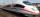German runways test a new ICE train between Munich and Berlin. The train runs to Berlin at a slow speed of 100 km/h. Back from Berlin goes faster. How quickly did the train have to go on a return trip so that the total average train speed for both journey
• Bus vs. trainBus started from point A 10 minutes before the train started from the same place. The bus went an average speed of 49 km/h, train 77 km/h. To point B train and bus arrived simultaneously. Calculate time of train journey, if train and bus travelled the sam
• Train and car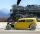The train and the car started at a constant speed to journey. When the train travels 87 km, the car travels 97 km. How many km travels the train when the car travels 87 km?
• Bus stop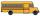Determine at what time the bus leaves from the bus stop outside the village, if you know that when you leave home at 8:00 and go at speed 3 km/h, you come to a stop 9 minutes after the departure of the bus, and when you go speed at 4 km/h, you come to the
• Average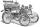What is the average speed of the car, where half of the distance covered passed at speed 66 km/h and the other half at 86 km/h.
• Motion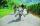Cyclist started at 9:00 from point S to point T. After 10 minutes, followed him at the same speed the second cyclist. Walker, which went from T to S, started at 9:35. After 71 minutes he met the first cyclist and after next 8 minutes the second cyclist. D
• TruckIn 7 hours started from town Krnov truck at speed 40 km/h. Passenger car started against it in 8 hours 30 minutes from the city of Jihlava at speed 70 km/h. Distance between this two cities is 225 km. At what time and at what distance from Krnov this two
• Car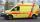Car goes some distance in 3 hours and 20 minutes. If it increase speed by 10 km/h, i goes this distance in 2.5 hours. Calculate the distance.